## Friday, February 13, 2009

### Irodov Problem 1.274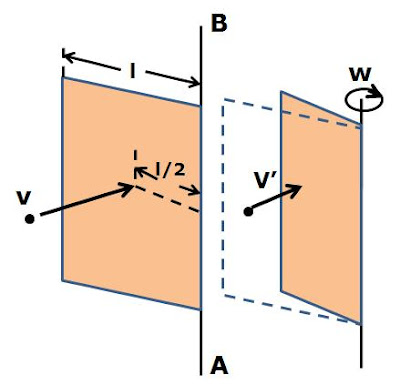a) Since the collision between the plate and the point mass is elastic, no energy is lost in the collision. Further, since there is no external torque acting on the (point mass + plate) system, the angular momentum of the (point mass + plate) system will be conserved.

Due to the symmetry of the collision it is clear that the point mass will continue to move along its initial direction of motion (either in the same direction or exactly opposite) after the collision. Suppose that the velocity of the point mass after collision be v'. Further suppose that the collision also results in the plate acquiring an angular velocity of w. The moment of inertia of the plate about axis AB will be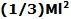(see this list). Conservation of angular momentum about the axis AB gives us,Conserving initial and final energies we have,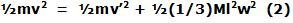From (1) and (2) we have,One trivial solution for (3) is v=v' which implies that the point mass simply passed through and through the plate without doing anything to it. Clearly this is no possible so we neglect it. The other solution gives us,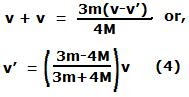Now using (1) and (4) we can find w as,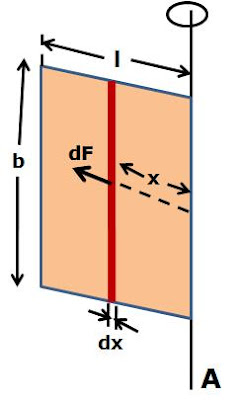Let the width of the plate be b and its surface density (mass/unit area) be p. Consider a infinitesimally thin vertical slice of the plate of thickness dx at a distance x from the axis. The mass of this slice is bpdx. Since it rotates at a distance x and with a angular velocity w it pulls the rest of the plate with a forceradially outwards. The total force on the axis is found by integrating over all such slices as,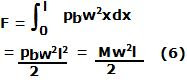From (5) and (6) we have,Since the plate is not flying out radially, this must be exactly euqal to the force exterted by the axis to support the rotating plate.